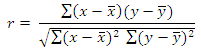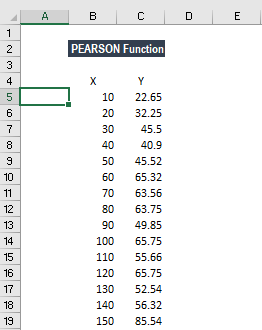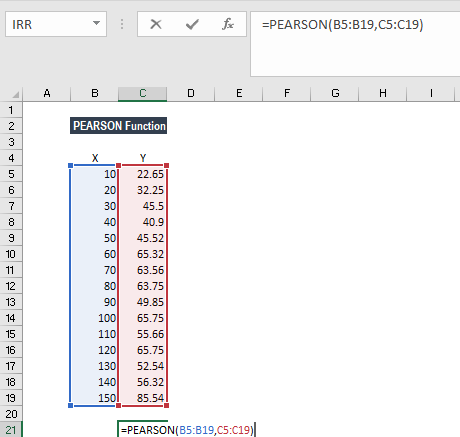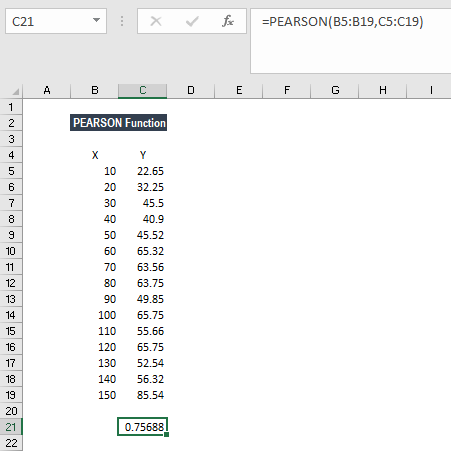# PEARSON Function

Calculates the Pearson Product-Moment Correlation Coefficient for two sets of values

## What is the PEARSON Function?

The PEARSON function is categorized under Excel Statistical functions. It will calculate the Pearson Product-Moment Correlation Coefficient for two sets of values. For example, we can find out the relationship between the age of a person and the appearance of grey hair.

As a financial analyst, the PEARSON function is useful in understanding the relationship between Earnings per Share (EPS) and other financial metrics.

### Formula

=PEARSON(array1, array2)

The PEARSON function uses the following arguments:

1. Array1 (required argument) – This is the number set of independent values.
2. Array2 (It is a required argument) – This is the set of dependent values.

The function ignores text values and logical values that are supplied as part of an array. The Pearson product-moment correlation coefficient for two sets of values, x and y, is given by the formula:Where x and y are the sample means of the two arrays of values.

If the value of r is close to +1, it indicates a strong positive correlation, and if r is close to -1, it denotes a strong negative correlation.

### How to use PEARSON Function in Excel?

To understand the uses of the PEARSON function, let’s consider an example:

#### Example

Suppose we are given the following sets of data and we wish to find out the correlation between them:The formula to use is:We get the result below:The result above implies a positive correlation among the data sets.

### Things to remember about the PEARSON Function

1. #N/A! error – Occurs if the given array arguments are of different lengths.
2. #DIV/0! error – Occurs when either of the given array arguments is empty or when the standard deviation of their values is equal to zero.
3. The PEARSON function performs the same calculation as the CORREL function. However, in MS Excel 2003 and earlier versions, PEARSON may exhibit some rounding errors.
4. If an array or reference argument contains text, logical values, or empty cells, the values are ignored. However, cells with the value zero are included by this function.

Thanks for reading CFI’s guide to important Excel functions! By taking the time to learn and master these functions, you’ll significantly speed up your financial modeling. To learn more, check out these additional CFI resources:

• Excel Functions for Finance